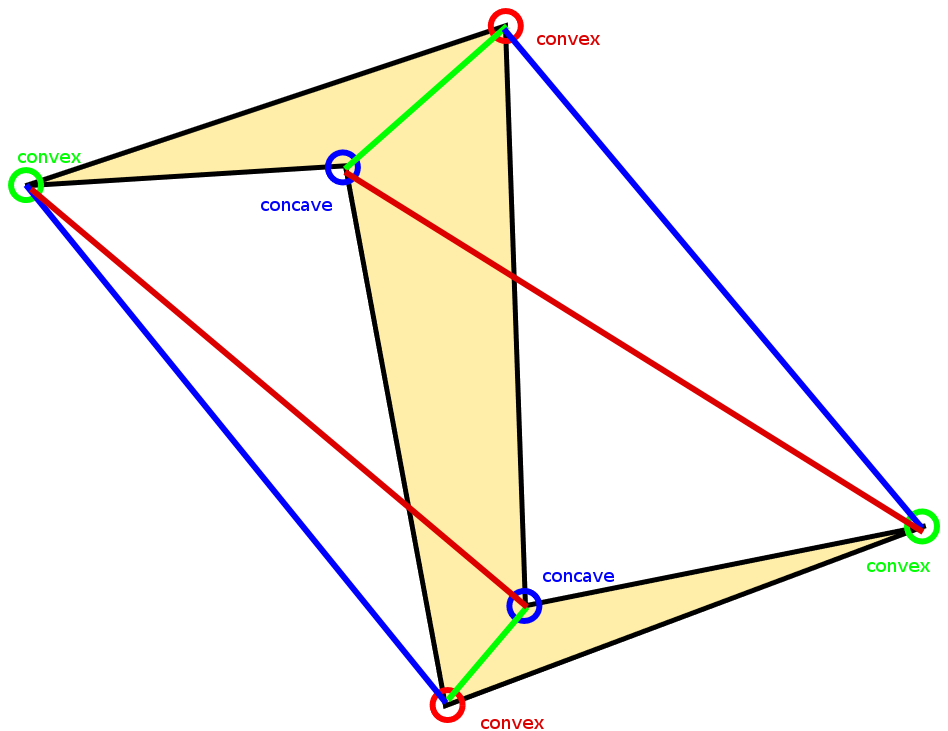20 Oct

The mathematical purpose of this project is to explore the geometry of the piles that form when sand is poured on different 2D shapes. Specifically, we investigate the ridges and apexes that form on these piles. In the case of the polygonal shapes, we define a ridge as where two planes meet at a line, and an apex as where three or more planes meet at a point. We decided to focus on these aspects, and particularly their projections onto the 2D shape beneath them because they are easier to analyze in 2D, and because the actual angle the pile of sand slopes at depends on the sand itself, and not on the geometry of the shape it is on.

First, we define what convex/concave means. Consider any vertex of a polygon. Then look at the two vertices at the ends of the two edges that form the original vertex. If the line drawn between these two vertices lies at least partially inside of the polygon, then define the original vertex as a convex vertex. If the line lies entirely outside of the polygon, then define the original vertex as a concave vertex.The main observation that we came to and hope that participants will gain intuition for is that, given a polygon, the ridges when projected onto the shape are the angle-bisectors of each convex vertex, and the apexes occur where these bisectors meet. Once they meet at an apex, the bisectors disappear and are replaced with a single new ridge that connects to another apex (this is particularly visible in the case of the rectangle). The logic behind this phenomenon is that a convex vertex produce ridges because sand resting on top of the shape needs to minimize the distance between its two forming edges, whereas with a concave vertex, its two forming edges create negative space where sand cannot rest.

Note that concave vertices do not create ridges or affect other ridges, which is exemplified by the the fact that the triangle and the concave hexagon have the same ridges. The logic behind this phenomenon is that a convex vertex produce ridges because sand resting on top of the shape needs to fall in the middle of two edges, whereas with a concave vertex, its two forming edges create negative space where sand cannot rest.

While the curved shapes appear to be more intimidating, they are in fact governed by the same principle: the “ridge” is the set of points equidistant to the two closet edges. In the cases of polygons, this set is the angle bisector.

In the case of the curved plates, this definition defines conic sections. For the circular plate with the circular hole in the center, the set of points exactly halfway between the two circles in a circle exactly halfway between the other two. For the off-center circle, this same process makes what looks like a circle in 2D, but is actually an ellipse in 3D. Lastly, the small hole on the rectangular plate simulates part of a parabola, since a parabola is defined to be the set of points equidistant to a point and and line (and here we approximate a point as a small circle, so sand can fall through).

## One thought on “Doing Math: The Math Behind Sand Piles”

1.Really cool! I wish as many people took the time to intuit the math behind this as they do look at the exhibit.